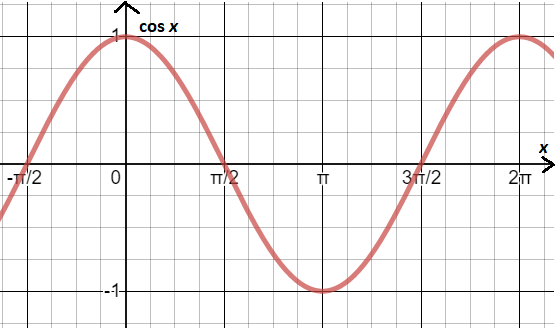QUESTION

# As the value of x increases from 0 to $\dfrac{\pi }{2}$, the value of $\cos x$:A. increasesB. decreasesC. remains constantD. increases, then decreases

Hint: Let us plot the graph for the trigonometric function $\cos x$. So, we can clearly see from that whether the value of x increases or decreases when x changes from 0 to $\dfrac{\pi }{2}$.So, some results that we get from the above graph were,
Change in value of the cosine function depends on the interval in which x lies.

So, when x belongs from 0 to $\pi$ (i.e. ${0^0}$ to ${180^0}$) then the value of the cosine function changes from 1 to – 1. Therefore $\cos x$ will be the decreasing function when x belongs from 0 to $\pi$.

And, when x belongs from $\pi$ to $2\pi$ (i.e. ${180^0}$ to ${360^0}$) then the value of the cosine function changes from – 1 to 1. Therefore $\cos x$ will be the increasing function when x belongs from $\pi$ to $2\pi$.
As we can see from the above graph that the value of $\cos x$ when the value of x is equal to 0 is 1.
And the value of $\cos x$ when the value of x is equal to $\dfrac{\pi }{2}$ is 0.
So, when the value of x increases from 0 to $\dfrac{\pi }{2}$, then the value of $\cos x$ decreases.
Hence, the correct option will be B.
Note:- Whenever we come up with this type of question where we are asked to find whether the trigonometric function is increasing or decreasing in the given specified range then we can also check that by putting different values of x in that trigonometric function and then calculating its value. From that we will get whether the value of the function increases or decreases when we increase the value of x from the lower limit of the range to the upper limit of the range.